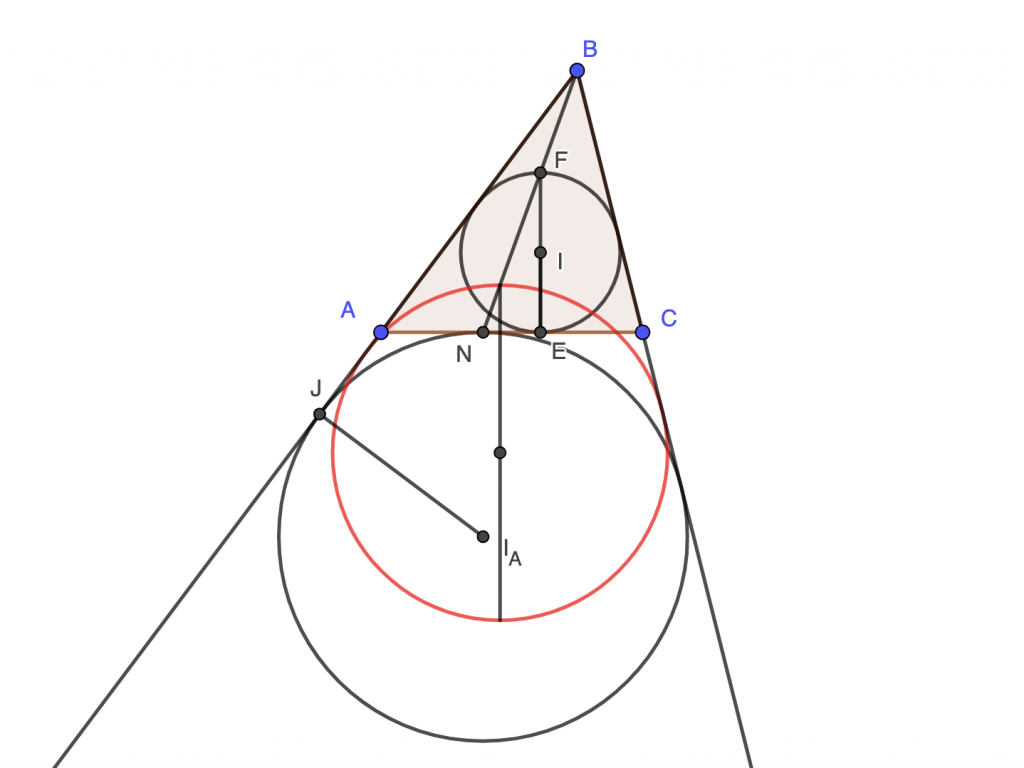How Cheenta works to ensure student success?
Explore the Back-Story

# Diameter of Incircle Lemma and Dilation of IncircleSuppose we have a triangle $ABC$. Let us extend the sides $BA$ and $BC$. We will draw the incircle of this triangle.

### How to draw the incircle?

• Here is the construction.
• Draw any two angle bisectors, say of angle $A$ and angle $B$
• Mark the intersection point $I$.
• Drop a perpendicular line from I to one of the sides, say $AC$ in this picture.
• Suppose the perpendicular intersects $AC$ at $E$.
• The incircle is drawn centred at $I$ and with radius $IE$

Suppose EI intersects the incircle at F.

### How to draw the excircle?

Now let us draw the excircle.

To do that we will need the angle bisector of external angle A and external angle C. Suppose they intersect at $I_A$. Drop a perpendicular from $I_A$ to extended $BA$ or extended $BC$ or $AC$. In this picture we drop it on extended $BA$ Suppose $J$ is the point of intersection of extended $BA$ and the perpendicular.

Draw a circle centred at $I_A$ and radius $I_A J$. This is the excircle.

### Dilating the incircle to excircle

The incircle can be dilated or blown up with respect to point $B$ into the excircle. The center $I$ is sent to the center $I_A$ under dilation $FE$ which is perpendicular to $AC$ is sent to another segment perpendicular to $AC$ as angles are preserved under dilation

### Questions:

• What is the ratio of dilation?
• How can you rigorously show that the center $I$ goes to the center $I_A$ under this dilation?
• Where do the point $F$ go under this dilation?
• Show that $AN = CE$

## Geogebra workbook

Suppose we have a triangle $ABC$. Let us extend the sides $BA$ and $BC$. We will draw the incircle of this triangle.

### How to draw the incircle?

• Here is the construction.
• Draw any two angle bisectors, say of angle $A$ and angle $B$
• Mark the intersection point $I$.
• Drop a perpendicular line from I to one of the sides, say $AC$ in this picture.
• Suppose the perpendicular intersects $AC$ at $E$.
• The incircle is drawn centred at $I$ and with radius $IE$

Suppose EI intersects the incircle at F.

### How to draw the excircle?

Now let us draw the excircle.

To do that we will need the angle bisector of external angle A and external angle C. Suppose they intersect at $I_A$. Drop a perpendicular from $I_A$ to extended $BA$ or extended $BC$ or $AC$. In this picture we drop it on extended $BA$ Suppose $J$ is the point of intersection of extended $BA$ and the perpendicular.

Draw a circle centred at $I_A$ and radius $I_A J$. This is the excircle.

### Dilating the incircle to excircle

The incircle can be dilated or blown up with respect to point $B$ into the excircle. The center $I$ is sent to the center $I_A$ under dilation $FE$ which is perpendicular to $AC$ is sent to another segment perpendicular to $AC$ as angles are preserved under dilation

### Questions:

• What is the ratio of dilation?
• How can you rigorously show that the center $I$ goes to the center $I_A$ under this dilation?
• Where do the point $F$ go under this dilation?
• Show that $AN = CE$

## Geogebra workbook

This site uses Akismet to reduce spam. Learn how your comment data is processed.

### Knowledge Partner